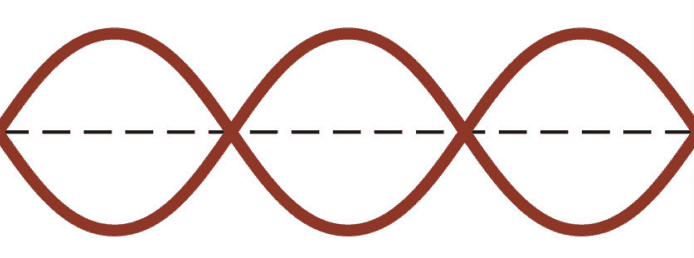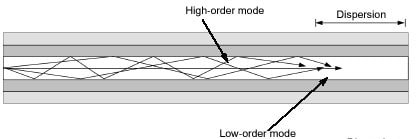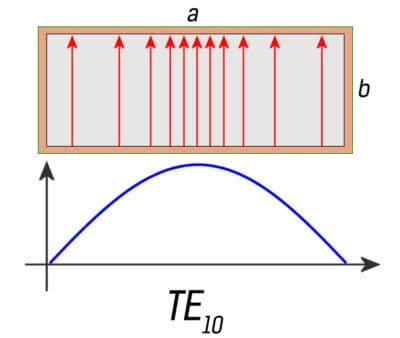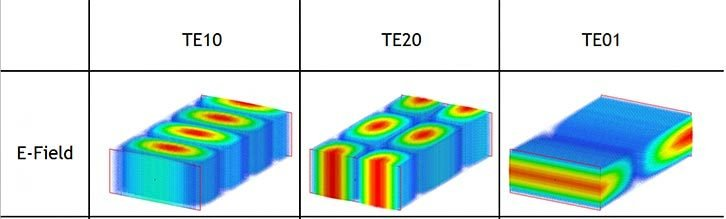# Optical Fiber Communication Basics – Mode theory of light

The Ray theory of light does not consider the wave nature of a light wave. So to better understand the behavior of light in an optical fiber, we use another theory called Mode theory.

Please note that mode theory is a part of the electromagnetic theory of light that we use to understand the behavior of light in an optical fiber cable. It does not have its own set of hypotheses.

Before we get started, let’s talk about waves. When two waves of the same frequency and amplitude traveling in opposite directions meet, they form a standing wave. The figure below shows a very basic version of this phenomenon. Whenever a standing wave is produced, there is no transfer of energy. The number of loops in this standing wave is called the order of the wave.### Why is it important to know that simple fact about a standing wave?

Optical fibers are meant to transfer optical energy. So the presence of a standing wave is not desirable since it won’t help in transferring energy.

## Understanding mode theory with the help of ray theory

Considering light as a simple ray to explain the physical manifestation of modes. Simply put, we will use ray theory to introduce you to mode theory. Modes are the patterns of electromagnetic waves in a waveguide. It is possible to have a vast number of modes (patterns) for every light signal passing through a waveguide. Low order modes travel without a lot of reflections. Higher order modes tend to reflect a lot. Due to these reflections, it is possible that standing waves may be generated, and standing waves are not good for energy propagation.Notice that lower order modes travel almost in an absolutely straight line, while higher order modes ‘bounce’ around quite a bit.

Mind that reflections are not bad. If the light signal has an angle greater than the critical angle and performs total internal reflections, it is not an issue. But as we go to higher modes, we start to notice losses, as light energy begins to be absorbed by the cladding and doesn’t get completely reflected on collision with the walls of the core.

Hence higher order modes are not desirable.

## Mode theory of an electromagnetic wave

Now to get in the thick of things, light has both electrical and magnetic components. If a light wave is moving in the z direction and if

1. there is no electric field component in the z direction (electric field is transverse to the z-axis), the wave is called Transverse Electric wave (TE wave).
2. Similarly, if there is no magnetic field component in the z direction ( the magnetic field is transverse to the z-axis), the wave is called Transverse Magnetic wave (TE wave).
3. If neither component (electric or magnetic) is moving in the z-direction, then the wave is called as Transverse ElectroMagnetic wave (TEM wave).

A few paragraphs above, we saw that modes could be thought of as all the possible patterns of a light wave in a waveguide. To make that definition more accurate, consider a waveguide with a breadth of ‘a’ units and width of ‘b’ units. Let m be the number of half waves of either electric or magnetic field across breadth a. Also, let n be the number of half waves of either electric or magnetic fields across width b. For any wave, the subscript mn will denote its mode. This might be tricky to understand, so let’s look at an example.

Consider the half wave above to be a transverse electric (TE) wave. The electric field goes from 0 to max and back to 0. Now take a look at the diagram below. It shows the cross section of a waveguide with the electric field lines. Since the variation is across breadth a, m = 1. So this mode of the TE wave will be represented as$TE_{10}$.### Field Pattern

You will encounter images similar to the above one throughout this course. A diagram which shows the variation of electric and magnetic field lines is called a field pattern.Some field patterns can be a bit more detailed like this one shows a heat map of the electrical field intensity of different modes.

## What should you remember?

In summary, to be crude, you can consider modes to be patterns of all possible waves. It’s not wrong but not completely accurate. Mode can be better defined by using the concept of variations in TE and TM waves across a waveguide. Higher order modes are not good for optical fiber communication. And as we will see in this course, there are techniques to make sure that losses arising from high mode signals are minimal.

This site uses Akismet to reduce spam. Learn how your comment data is processed.